# 1.2.2: Aliphatic Hydrocarbons

$$\newcommand{\vecs}{\overset { \rightharpoonup} {\mathbf{#1}} }$$ $$\newcommand{\vecd}{\overset{-\!-\!\rightharpoonup}{\vphantom{a}\smash {#1}}}$$$$\newcommand{\id}{\mathrm{id}}$$ $$\newcommand{\Span}{\mathrm{span}}$$ $$\newcommand{\kernel}{\mathrm{null}\,}$$ $$\newcommand{\range}{\mathrm{range}\,}$$ $$\newcommand{\RealPart}{\mathrm{Re}}$$ $$\newcommand{\ImaginaryPart}{\mathrm{Im}}$$ $$\newcommand{\Argument}{\mathrm{Arg}}$$ $$\newcommand{\norm}{\| #1 \|}$$ $$\newcommand{\inner}{\langle #1, #2 \rangle}$$ $$\newcommand{\Span}{\mathrm{span}}$$ $$\newcommand{\id}{\mathrm{id}}$$ $$\newcommand{\Span}{\mathrm{span}}$$ $$\newcommand{\kernel}{\mathrm{null}\,}$$ $$\newcommand{\range}{\mathrm{range}\,}$$ $$\newcommand{\RealPart}{\mathrm{Re}}$$ $$\newcommand{\ImaginaryPart}{\mathrm{Im}}$$ $$\newcommand{\Argument}{\mathrm{Arg}}$$ $$\newcommand{\norm}{\| #1 \|}$$ $$\newcommand{\inner}{\langle #1, #2 \rangle}$$ $$\newcommand{\Span}{\mathrm{span}}$$

Learning Objectives

• Define and identify alkanes, alkenes, alkynes, and cyclic hydrocarbons.
• List some properties of hydrocarbons.
• Identify and name simple (straight-chain) alkanes given formulas and write formulas for straight-chain alkanes given their names.
• Define structural and geometric isomers.
• Write condensed structural formulas and draw line-angle formulas given complete structural formulas for alkanes,alkenes, alkynes, and cyclic hydrocarbons.

The simplest organic compounds are those composed of only two elements: carbon and hydrogen. These compounds are called hydrocarbons. Hydrocarbons themselves are separated into two types: aliphatic hydrocarbons and aromatic hydrocarbons. Aliphatic hydrocarbons are hydrocarbons based on chains of C atoms. There are three types of aliphatic hydrocarbons. Alkanes are aliphatic hydrocarbons with only single covalent bonds. Alkenes are hydrocarbons that contain at least one C–C double bond, and alkynes are hydrocarbons that contain a C–C triple bond. Occasionally, we find an aliphatic hydrocarbon with a ring of C atoms; these hydrocarbons are called cycloalkanes (or cycloalkenes or cycloalkynes). Because alkanes have the maximum number of H atoms possible according to the rules of covalent bonds, alkanes are also referred to as saturated hydrocarbons.

Note

The word saturated has the same meaning for hydrocarbons as it does for the dietary fats and oils: the molecule has no carbon-to-carbon double bonds (C=C).

## Alkanes

The simplest alkanes have their C atoms bonded in a straight chain; these are called normal alkanes. They are named according to the number of C atoms in the chain. The smallest alkane is methane:Figure $$\PageIndex{1}$$.Figure $$\PageIndex{1}$$ Three-dimensional representation of methane © Thinkstock. The methane molecule is three dimensional, with the H atoms in the positions of the four corners of a tetrahedron.

The diagrams representing alkanes are called structural formulas because they show the structure of the molecule. As molecules get larger, structural formulas become more and more complex. One way around this is to use a condensed structural formula, which lists the formula of each C atom in the backbone of the molecule.

condensed structural formula

The condensed formulas show hydrogen atoms right next to the carbon atoms to which they are attached, as illustrated for butane:The ultimate condensed formula is a line-angle formula (or line drawing) , in which carbon atoms are implied at the corners and ends of lines, and each carbon atom is understood to be attached to enough hydrogen atoms to give each carbon atom four bonds. For example, we can represent pentane (CH3CH2CH2CH2CH3) and isopentane [(CH3)2CHCH2CH3] as follows:Structural FormulaBall and Stick Model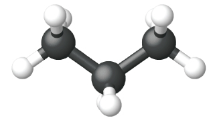Space Filling model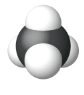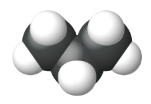Name Methane Ethane Propane Condensed Structural Formula CH4 CH3CH3 CH3CH2CH3 Molecular Formula CH4 C2H6 C3H8 Line angle drawing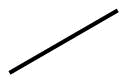Example $$\PageIndex{1}$$

Provide the molecular formula, the complete structural formula, the condensed structural formula and the line angle formula for a straight chain alkane with 8 carbon atoms.

Solutions

Molecular Formula

The general formula for an alkane is CnH2n + 2

For an alkane with 8 carbon atoms, C8H(2 × 8) + 2 = C8H18.

C8H18.​​​​​​
Complete Structural Formula Eight carbons first linked together and all remaining bonds to hydrogen to fulfill the octet rule.

C1-C2-C3-C4-C5-C6-C7-C8

Condensed Structural Formula

The terminal carbon atoms (C1 and C8) are bonded to three hydrogen atoms each and the six middle carbon atoms are bonded to two hydrogen atoms each.

The six middle carbon atoms are all bonded to two hydrogen atoms so the structural formula can be simplified further.

CH3CH2CH2CH2CH2CH2CH2CH3

CH3(CH2)6CH3

Line Angle Drawing

Eight carbon atoms are implied at the corners and ends of lines, and each carbon atom is understood to be attached to enough hydrogen atoms to give each carbon atom four bonds.

Exercise $$\PageIndex{1}$$

Provide the molecular formula, the complete structural formula, the condensed structural formula, and the line angle formula for a straight chain alkane with 7 carbon atoms.

## Homologous Series

Methane (CH4), ethane (C2H6), and propane (C3H8) are the beginning of a series of compounds in which any two members in a sequence differ by one carbon atom and two hydrogen atoms—namely, a CH2 unit. The first 10 members of this series are given in Table $$\PageIndex{1}$$.

Table $$\PageIndex{1}$$ The First 10 Straight-Chain Alkanes
Molecular Formula Condensed Structural Formula Name
CH4 CH4 methane
C2H6 CH3CH3 ethane
C3H8 CH3CH2CH3 propane
C4H10 CH3CH2CH2CH3 butane
C5H12 CH3CH2CH2CH2CH3 pentane
C6H14 CH3(CH2)4CH3 hexane
C7H16 CH3(CH2)5CH3 heptane
C8H18 CH3(CH2)6CH3 octane
C9H20 CH3(CH2)7CH3 nonane
C10H22 CH3(CH2)8CH3 decane

Note

Parentheses in condensed structural formulas indicate that the enclosed grouping of atoms is attached to the adjacent carbon atom.

Consider the series in Figure $$\PageIndex{2}$$. The sequence starts with C3H8, and a CH2 unit is added in each step moving up the series. Any family of compounds in which adjacent members differ from each other by a definite factor (here a CH2 group) is called a homologous series. The members of such a series, called homologs, have properties that vary in a regular and predictable manner. The principle of homology gives organization to organic chemistry in much the same way that the periodic table gives organization to inorganic chemistry. Instead of a bewildering array of individual carbon compounds, we can study a few members of a homologous series and from them deduce some of the properties of other compounds in the series.Figure $$\PageIndex{2}$$ Members of a homologous series.

The principle of homology allows us to write a general formula for alkanes: CnH2n + 2. Using this formula, we can write a molecular formula for any alkane with a given number of carbon atoms. For example, an alkane with eight carbon atoms has the molecular formula C8H(2 × 8) + 2 = C8H18.

### Naming (IUPAC Nomenclature)

A systematic way of naming hydrocarbons and other organic compounds has been devised by the International Union of Pure and Applied Chemistry (IUPAC). These rules, used worldwide, are known as the IUPAC System of Nomenclature. A stem name in Table $$\PageIndex{1}$$ indicates the number of carbon atoms in the longest continuous chain (LCC). Atoms or groups attached to this carbon chain, called substituents, are then named, with their positions indicated by numbers.

Table $$\PageIndex{2}$$Stems That Indicate the Number of Carbon Atoms in Organic Molecules
Stem Number of C Atoms
meth- 1
eth- 2
prop- 3
but- 4
pent- 5
hex- 6
hept- 7
oct- 8
non- 9
dec- 10

Note

A continuous (unbranched) chain of carbon atoms is often called a straight chain even though the tetrahedral arrangement about each carbon gives it a zigzag shape. Straight-chain alkanes are sometimes called normal alkanes, and their names are given the prefix n-. For example, butane is called n-butane. We will not use that prefix here because it is not a part of the system established by the International Union of Pure and Applied Chemistry.

Example $$\PageIndex{2}$$

What is the name of an eight carbon alkane?

Solution

The stem that refers to eight carbon atoms is oct plus the suffix -ane for an alkane, so the answer is Octane.

Exercise $$\PageIndex{2}$$

Provide the name for the following compounds:

a. seven carbon alkane

b. six carbon alkane

a. heptane

b. hexane

## Isomerism-Branched Chain Alkanes

One of the interesting aspects of organic chemistry is that it is three-dimensional. A molecule can have a shape in space that may contribute to its properties. Molecules can differ in the way the atoms are arranged - the same combination of atoms can be assembled in more than one way. These compounds are known as isomers. Isomers are molecules with the same molecular formulas, but different arrangements of atoms. We will look at some isomer possibilities for alkanes and alkenes.

We can write the structure of butane (C4H10) by stringing four carbon atoms in a row,

–C–C–C–C–

and then adding enough hydrogen atoms to give each carbon atom four bonds:The compound butane has this structure, but there is another way to put 4 carbon atoms and 10 hydrogen atoms together. Place 3 of the carbon atoms in a row and then branch the fourth one off the middle carbon atom:Now we add enough hydrogen atoms to give each carbon four bonds.There is a hydrocarbon that corresponds to this structure, which means that two different compounds have the same molecular formula: C4H10. The two compounds have different properties—for example, one boils at −0.5°C; the other at −11.7°C. Different compounds having the same molecular formula are called isomers.Figure $$\PageIndex{3}$$ Butane and isobutane. The ball-and-stick models of these two compounds show them to be isomers; both have the molecular formula C4H10.

Notice that C4H10 is depicted with a bent chain in Figure $$\PageIndex{3}$$. The four-carbon chain may be bent in various ways because the groups can rotate freely about the C–C bonds. However, this rotation does not change the identity of the compound. It is important to realize that bending a chain does not change the identity of the compound; all of the following represent the same compound, butane:The structure of isobutane shows a continuous chain of three carbon atoms only, with the fourth attached as a branch off the middle carbon atom of the continuous chain, which is different from the structures of butane (compare the two structures in Figure $$\PageIndex{3}$$.

Unlike C4H10, the compounds methane (CH4), ethane (C2H6), and propane (C3H8) do not exist in isomeric forms because there is only one way to arrange the atoms in each formula so that each carbon atom has four bonds.

Next beyond C4H10 in the homologous series is pentane. Each compound has the same molecular formula: C5H12. The compound at the far left is pentane because it has all five carbon atoms in a continuous chain. The compound in the middle is isopentane; like isobutane, it has a one CH3 branch off the second carbon atom of the continuous chain. The compound at the far right, discovered after the other two, was named neopentane (from the Greek neos, meaning “new”). Although all three have the same molecular formula, they have different properties, including boiling points: pentane, 36.1°C; isopentane, 27.7°C; and neopentane, 9.5°C.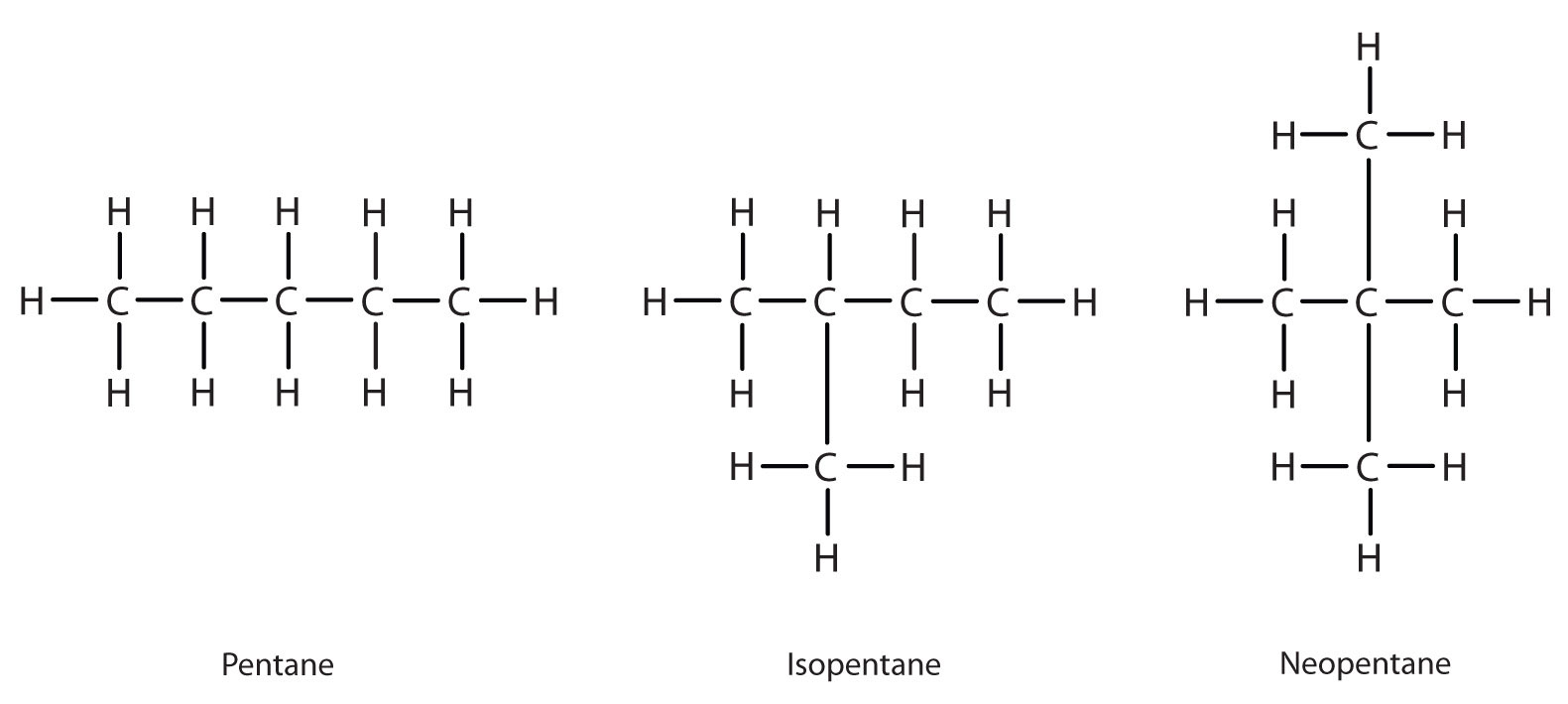### Naming Branched Alkanes

You have already learned the basics of nomenclature-the names of the first 10 normal hydrocarbons. Here, we will add some steps to the procedure so you can name branched hydrocarbons.

First, given the structure of an alkane, identify the longest continuous chain of C atoms. Note that the longest chain may not be drawn in a straight line. The longest chain determines the parent name of the hydrocarbon. For example, in the moleculethe longest chain of carbons has six C atoms. Therefore, it will be named as a hexane. However, in the molecule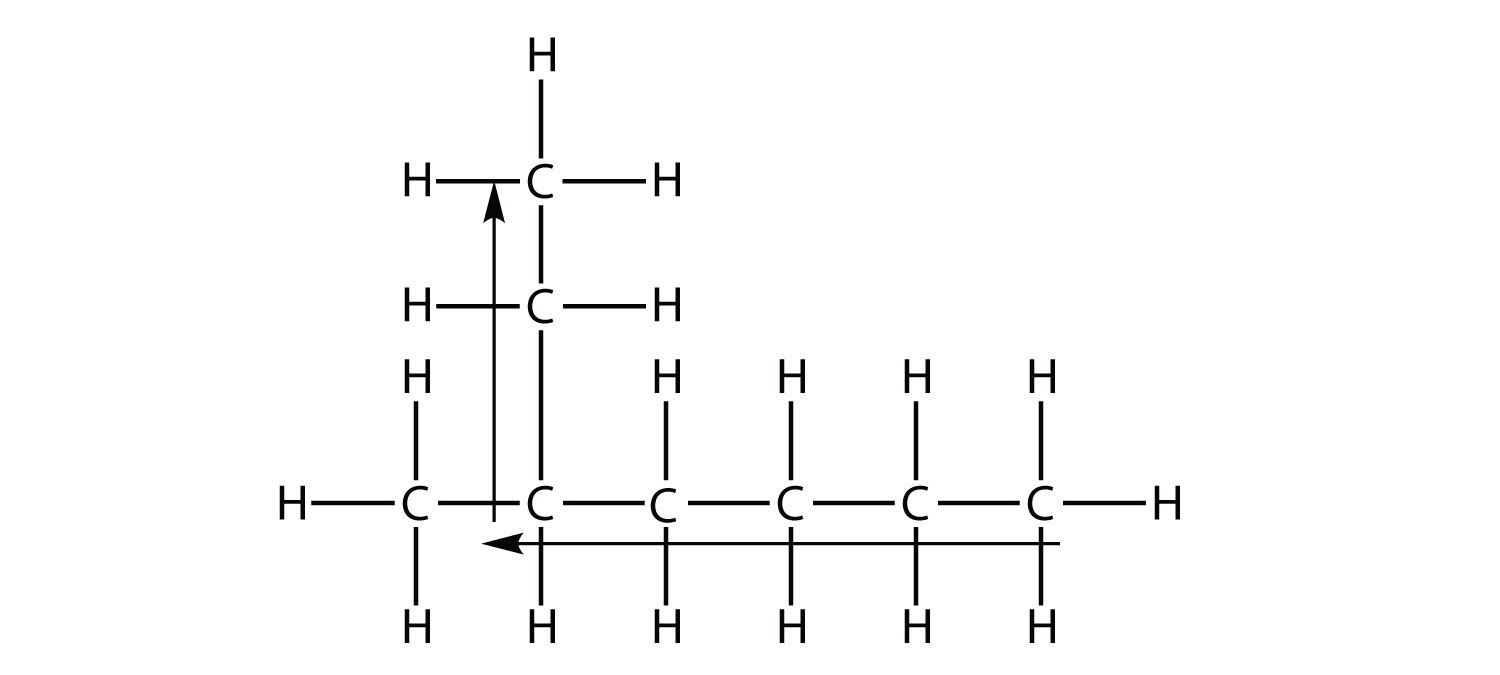the longest chain of C atoms is not six, but seven, as shown. So this molecule will be named as a heptane.

The next step is to identify the branches, or substituents, on the main chain. The names of the substituents, or alkyl groups, are derived from the names of the parent hydrocarbons; however, rather than having the ending -ane, the substituent name has the ending -yl. Table $$\PageIndex{3}$$, lists the substituent names for the five smallest substituents.

Table $$\PageIndex{3}$$ Substituent Names.

Substituent Formula Number of C Atoms Name of Substituent
CH3 1 methyl-
CH3CH2 2 ethyl-
CH3CH2CH2 3 propyl-
CH3CH2CH2CH2 4 butyl-
CH3CH2CH2CH2CH2 5 pentyl-
and so forth and so forth and so forth

In naming the branched hydrocarbon, the name of the substituent is combined with the parent name of the hydrocarbon without spaces. However, there is likely one more step. The longest chain of the hydrocarbon must be numbered, and the numerical position of the substituent must be included to account for possible isomers. As with double and triple bonds, the main chain is numbered to give the substituent the lowest possible number. For example, in this alkanethe longest chain is five C atoms long, so it is a pentane. There is a one-carbon substituent on the third C atom, so there is a methyl group at position 3. We indicate the position using the number, which is followed by a hyphen, the substituent name, and the parent hydrocarbon name-in this case, 3-methylpentane. That name is specific to that particular hydrocarbon and no other molecule. Organic chemistry nomenclature is very specific!

It is common to write the structural formula of a hydrocarbon without the H atoms, for clarity. So we can also represent 3-methylpentane aswhere it is understood that any unwritten covalent bonds are bonds with H atoms. With this understanding, we recognize that the structural formula for 3-methylpentane refers to a molecule with the formula of C6H14.

Example $$\PageIndex{3}$$:

Name this molecule.Solution

The longest continuous carbon chain has seven C atoms, so this molecule will be named as a heptane. There is a two-carbon substituent on the main chain, which is an ethyl group. To give the substituent the lowest numbering, we number the chain from the right side and see that the substituent is on the third C atom. So this hydrocarbon is 3-ethylheptane.

Exercise $$\PageIndex{3}$$

Name this molecule.2-methylpentane

Branched hydrocarbons may have more than one substituent. If the substituents are different, then give each substituent a number (using the smallest possible numbers) and list the substituents in alphabetical order, with the numbers separated by hyphens and with no spaces in the name. So the moleculeis 3-ethyl-2-methylpentane.

If the substituents are the same, then use the name of the substituent only once, but use more than one number, separated by a comma. Also, put a numerical prefix before the substituent name that indicates the number of substituents of that type. The numerical prefixes are listed in Table $$\PageIndex{4}$$. The number of the position values must agree with the numerical prefix before the substituent.

Table $$\PageIndex{4}$$ Numerical Prefixes to Use for Multiple Substituents
Number of Same Substituent Numerical Prefix
2 di-
3 tri-
4 tetra-
5 penta-
and so forth and so forth

Consider this molecule:The longest chain has four C atoms, so it is a butane. There are two substituents, each of which consists of a single C atom; they are methyl groups. The methyl groups are on the second and third C atoms in the chain (no matter which end the numbering starts from), so we would name this molecule 2,3-dimethylbutane. Note the comma between the numbers, the hyphen between the numbers and the substituent name, and the presence of the prefix di- before the methyl. Other molecules-even with larger numbers of substituents-can be named similarly.

Example $$\PageIndex{4}$$:

Name this molecule.Solution

The longest chain has seven C atoms, so we name this molecule as a heptane. We find two one-carbon substituents on the second C atom and a two-carbon substituent on the third C atom. So this molecule is named 3-ethyl-2,2-dimethylheptane.

Exercise $$\PageIndex{4}$$

Name this molecule.4,4,5-tripropyloctane

## Properties of Alkanes

All alkanes are composed of carbon and hydrogen atoms, and have similar bonds, structures, and formulas; noncyclic alkanes all have a formula of CnH2n+2. The number of carbon atoms present in an alkane has no limit. Greater numbers of atoms in the molecules will lead to stronger intermolecular attractions (dispersion forces) and correspondingly different physical properties of the molecules. Properties such as melting point and boiling point (Table $$\PageIndex{5}$$) usually change smoothly and predictably as the number of carbon and hydrogen atoms in the molecules change.

Table $$\PageIndex{5}$$ Properties of Some Alkanes
Alkane Molecular Formula Melting Point (°C) Boiling Point (°C) Phase at STP4 Number of Structural Isomers
methane CH4 –182.5 –161.5 gas 1
ethane C2H6 –183.3 –88.6 gas 1
propane C3H8 –187.7 –42.1 gas 1
butane C4H10 –138.3 –0.5 gas 2
pentane C5H12 –129.7 36.1 liquid 3
hexane C6H14 –95.3 68.7 liquid 5
heptane C7H16 –90.6 98.4 liquid 9
octane C8H18 –56.8 125.7 liquid 18
nonane C9H20 –53.6 150.8 liquid 35
decane C10H22 –29.7 174.0 liquid 75
tetradecane C14H30 5.9 253.5 solid 1858
octadecane C18H38 28.2 316.1 solid 60,523

Alkanes are relatively stable molecules, but heat or light will activate reactions that involve the breaking of C–H or C–C single bonds. Combustion is one such reaction:

$\ce{CH4}(g)+\ce{2O2}(g)⟶\ce{CO2}(g)+\ce{2H2O}(g)$

Alkanes burn in the presence of oxygen, a highly exothermic oxidation-reduction reaction that produces carbon dioxide and water. As a consequence, alkanes are excellent fuels. For example, methane, CH4, is the principal component of natural gas. Butane, C4H10, used in camping stoves and lighters is an alkane. Gasoline is a liquid mixture of continuous- and branched-chain alkanes, each containing from five to nine carbon atoms, plus various additives to improve its performance as a fuel. Kerosene, diesel oil, and fuel oil are primarily mixtures of alkanes with higher molecular masses. The main source of these liquid alkane fuels is crude oil, a complex mixture that is separated by fractional distillation. Fractional distillation takes advantage of differences in the boiling points of the components of the mixture (Figure $$\PageIndex{4}$$). You may recall that boiling point is a function of intermolecular interactions, which was discussed in the chapter on solutions and colloids.Figure $$\PageIndex{4}$$ In a column for the fractional distillation of crude oil, oil heated to about 425 °C in the furnace vaporizes when it enters the base of the tower. The vapors rise through bubble caps in a series of trays in the tower. As the vapors gradually cool, fractions of higher, then of lower, boiling points condense to liquids and are drawn off. (credit left: modification of work by Luigi Chiesa)

In a substitution reaction, another typical reaction of alkanes, one or more of the alkane’s hydrogen atoms is replaced with a different atom or group of atoms. No carbon-carbon bonds are broken in these reactions, and the hybridization of the carbon atoms does not change. For example, the reaction between ethane and molecular chlorine depicted here is a substitution reaction:The C–Cl portion of the chloroethane molecule is an example of a functional group, the part or moiety of a molecule that imparts a specific chemical reactivity. The types of functional groups present in an organic molecule are major determinants of its chemical properties and are used as a means of classifying organic compounds as detailed in the remaining sections of this chapter.

## Cyclic Hydrocarbons

A cyclic hydrocarbon is a hydrocarbon in which the carbon chain joins to itself in a ring. A cycloalkane is a cyclic hydrocarbon in which all of the carbon-carbon bonds are single bonds. Like other alkanes, cycloalkanes are saturated compounds. Cycloalkanes have the general formula $$\ce{C_{n}H_{2n}}$$. The simplest cycloalkane shown below is cyclopropane, a three-carbon ring.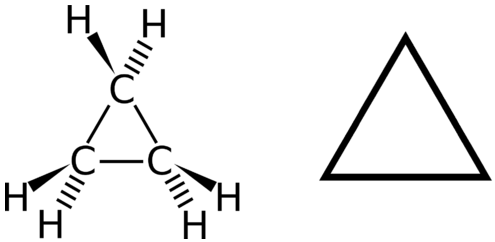Figure $$\PageIndex{5}$$ Cyclopropane the simplest cycloalkane.

The structural formulas of cyclic hydrocarbons can be represented in multiple ways, two of which are shown above. Each atom can be shown as in the structure on the left from the figure above. A convenient shorthand is to omit the element symbols and only show the shape, as in the triangle on the right. Carbon atoms are understood to be the vertices of the triangle.

The carbon atoms in cycloalkanes are still $$sp^3$$ hybridized, with an ideal bond angle of $$109.5^\text{o}$$. However, an examination of the cyclopropane structure shows that the triangular structure results in a $$\ce{C-C-C}$$ bond angle of $$60^\text{o}$$. This deviation from the ideal angle is called ring strain and makes cyclopropane a fairly unstable and reactive molecule. Ring strain is decreased for cyclobutane, with a bond angle of $$90^\text{o}$$, but is still significant. Cyclopentane has a bond angle of about $$108^\text{o}$$. This minimal ring strain for cyclopentane makes it a more stable compound.Cyclohexane is a six-carbon cycloalkane shown belowAll three of the depictions of cyclohexane are somewhat misleading because the molecule is not planar. In order to reduce the ring strain and attain a bond angle of approximately $$109.5^\text{o}$$, the molecule is puckered. The puckering of the ring means that every other carbon atom is above and below the plane. The figure below shows two possibilities for the puckered cyclohexane molecule. Each of the structures is called a conformation. The conformation on the right is called the boat conformation, while the one on the left is called the chair conformation.Figure $$\PageIndex{6}$$ Chair (left) and boat (right) conformations for cyclohexane.

While both conformations reduce the ring strain compared to a planar molecule, the chair is preferred. This is because the chair conformation results in fewer repulsive interactions between the hydrogen atoms. However, interconversion readily occurs between the two conformations.

Larger cycloalkanes also exist, but are less common. Cyclic hydrocarbons may also be unsaturated. A cycloalkene is a cyclic hydrocarbon with at least one carbon-carbon double bond. A cycloalkyne is a cyclic hydrocarbon with at least one carbon-carbon triple bond. Shown below are the simplified structural formulas for cyclohexene and cyclooctyne.## Unsaturated Hydrocarbons: Alkenes and Alkynes

### Alkenes

Organic compounds that contain one or more double or triple bonds between carbon atoms are described as unsaturated. Unsaturated hydrocarbons have less than the maximum number of H atoms possible. Unsaturated hydrocarbon molecules that contain one or more double bonds are called alkenes. Carbon atoms linked by a double bond are bound together by two bonds, one σ bond and one π bond. Double and triple bonds give rise to a different geometry around the carbon atom that participates in them, leading to important differences in molecular shape and properties. The differing geometries are responsible for the different properties of unsaturated versus saturated fats.

Note

The word unsaturated has the same meaning for hydrocarbons as it does for the dietary fats and oils: the molecule has at least one carbon-to-carbon double (C=C) or triple bond (C=C).

Ethene, C2H4, is the simplest alkene. Each carbon atom in ethene, commonly called ethylene, has a trigonal planar structure. The second member of the series is propene (propylene) (Figure $$\PageIndex{6}$$); the butene isomers follow in the series. Four carbon atoms in the chain of butene allows for the formation of isomers based on the position of the double bond, as well as a new form of isomerism.

 Structural FormulaBall and Stick Model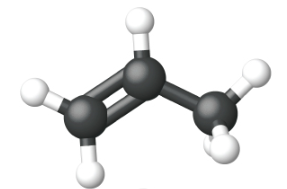Space Filling model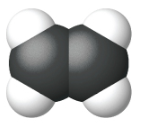Name ethene propene 1-butene Condensed Structural Formula CH2CH2 CH2CH​​​​​​CH3 CH3CH2CHCH2 Molecular Formula C2H2 C3H6 C4H8 Line angle drawingThe name of an alkene is derived from the name of the alkane with the same number of carbon atoms. The presence of the double bond is signified by replacing the suffix -ane with the suffix -ene. The location of the double bond is identified by naming the smaller of the numbers of the carbon atoms participating in the double bond:### Alkynes

Hydrocarbon molecules with one or more triple bonds are called alkynes; they make up another series of unsaturated hydrocarbons. Two carbon atoms joined by a triple bond are bound together by one σ bond and two π bonds. The sp-hybridized carbons involved in the triple bond have bond angles of 180°, giving these types of bonds a linear, rod-like shape.

The simplest member of the alkyne series is ethyne, C2H2, commonly called acetylene. The Lewis structure for ethyne, a linear molecule, is:The IUPAC nomenclature for alkynes is similar to that for alkenes except that the suffix -yne is used to indicate a triple bond in the chain. For example, $$\mathrm{CH_3CH_2C≡CH}$$ is called 1-butyne.

### Naming Alkenes and Alkynes

Alkenes and alkynes are named in a similar fashion. The biggest difference is that when identifying the longest carbon chain, it must contain the C–C double or triple bond. Furthermore, when numbering the main chain, the double or triple bond gets the lowest possible number. This means that there may be longer or higher-numbered substituents than may be allowed if the molecule were an alkane. For example, this moleculeis 2,4-dimethyl-3-heptene (note the number and the hyphens that indicate the position of the double bond).

Example $$\PageIndex{5}$$

Name this molecule.Solution

The longest chain that contains the C–C triple bond has six C atoms, so this is a hexyne molecule. The triple bond starts at the third C atom, so this is a 3-hexyne. Finally, there are two methyl groups on the chain; to give them the lowest possible number, we number the chain from the left side, giving the methyl groups the second position. So the name of this molecule is 2,2-dimethyl-3-hexyne.

Exercise $$\PageIndex{5}$$

Name this molecule.2,3,4-trimethyl-2-pentene

Example $$\PageIndex{6}$$

Identify the molecule as an alkane, an alkene, or an alkyne.

1.2.3.Solution

1. The molecule has at least one carbon-to-carbon triple bond (C=C) so it is an alkyne.

2. The molecule has a one carbon-to-carbon double bond (C=C) so it is an alkene.

3. The molecule only has carbon-to-carbon single bonds so it is an alkane.

Exercise $$\PageIndex{6}$$

Identify the molecule as an alkane, an alkene, or an alkyne.

1.2.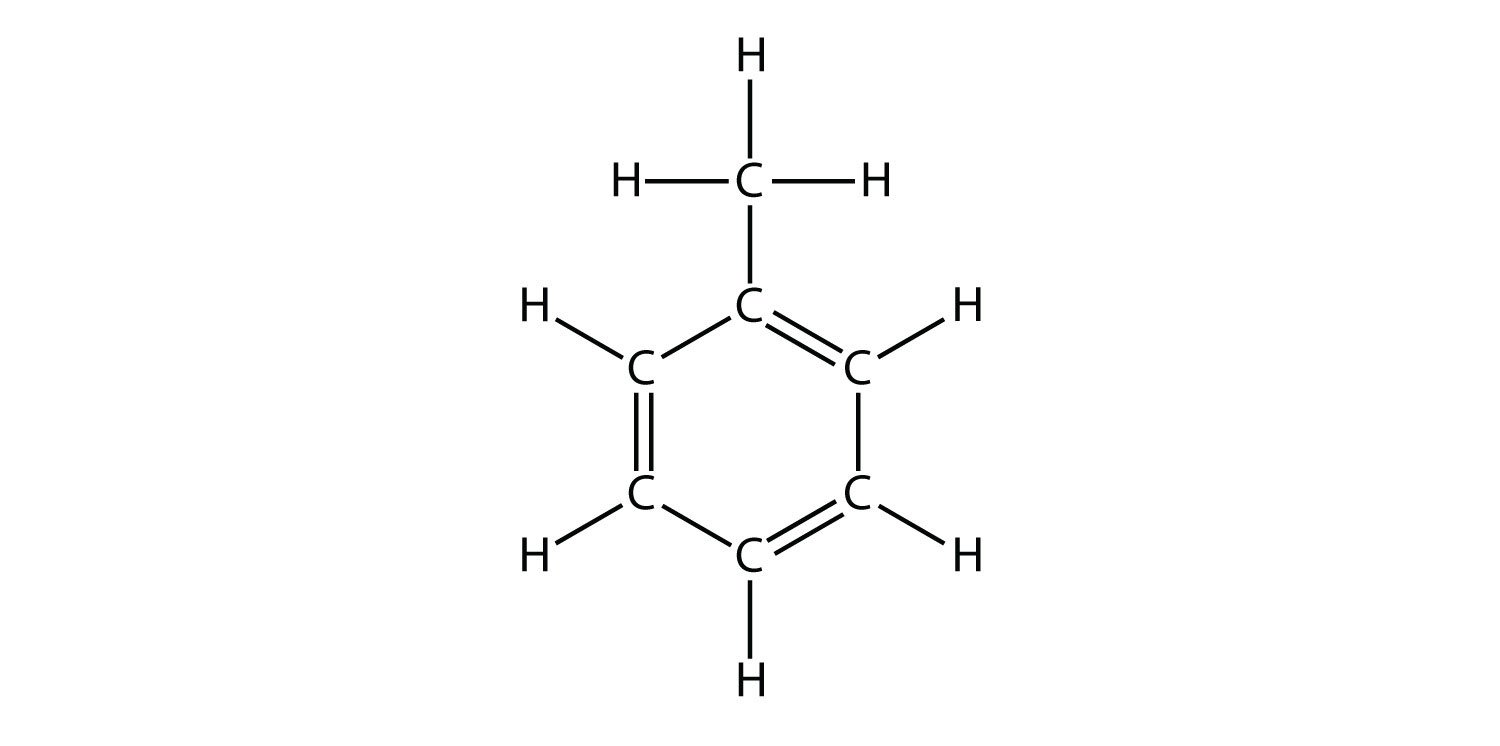3.1. alkane, 2. alkene, 3. alkyne

## Properties of Alkenes and Alkynes

Ethylene (the common industrial name for ethene) is a basic raw material in the production of polyethylene and other important compounds. Over 135 million tons of ethylene were produced worldwide in 2010 for use in the polymer, petrochemical, and plastic industries. Ethylene is produced industrially in a process called cracking, in which the long hydrocarbon chains in a petroleum mixture are broken into smaller molecules.

Halogens can also react with alkenes and alkynes, but the reaction is different. In these cases, the halogen reacts with the C–C double or triple bond and inserts itself onto each C atom involved in the multiple bonds. This reaction is called an addition reaction. One example isThe reaction conditions are usually mild; in many cases, the halogen reacts spontaneously with an alkene or an alkyne.

Hydrogen can also be added across a multiple bond; this reaction is called a hydrogenation reaction. In this case, however, the reaction conditions may not be mild; high pressures of H2 gas may be necessary. A platinum or palladium catalyst is usually employed to get the reaction to proceed at a reasonable pace:

$CH_{2}=CH_{2}+H_{2}\overset{metal\: catalyst}{\rightarrow} CH_{3}CH_{3}$

## Geometric Isomers (Cis-Trans) Isomers

With a molecule such as 2-butene, a different type of isomerism called geometric isomerism can be observed. Geometric isomers are isomers in which the order of atom bonding is the same but the arrangement of atoms in space is different. The double bond in an alkene is not free to rotate because of the nature of the pi bond. Therefore, there are two different ways to construct the 2-butene molecule. The image below shows the two geometric isomers, called cis-2-butene and trans-2-butene.The cis isomer has the two single hydrogen atoms on the same side of the molecule, while the trans isomer has them on opposite sides of the molecule. In both molecules, the bonding order of the atoms is the same. In order for geometric isomers to exist, there must be a rigid structure in the molecule to prevent free rotation around a bond. If the double bond in an alkene was capable of rotating, the two geometric isomers above would not exist. In addition, the two carbon atoms must each have two different groups attached in order for there to be geometric isomers. Propene has no geometric isomers because one of the carbon atoms has two single hydrogens bonded to it.Physical and chemical properties of geometric isomers are generally different. While cis-2-butene is a polar molecule, trans-2-butene is nonpolar. Heat or irradiation with light can be used to bring about the conversion of one geometric isomer to another. The input of energy must be large enough to break the pi bond between the two carbon atoms, which is weaker than the sigma bond. At that point,the now single bond is free to rotate and the isomers can interconvert.

As with alkenes, alkynes display structural isomerism beginning with 1-butyne and 2-butyne. However, there are no geometric isomers with alkynes because there is only one other group bonded to the carbon atoms that are involved in the triple bond.

## Summary

• Simple alkanes exist as a homologous series, in which adjacent members differ by a CH2 unit.
• Condensed chemical formulas show the hydrogen atoms (or other atoms or groups) right next to the carbon atoms to which they are attached.
• Line-angle formulas imply a carbon atom at the corners and ends of lines. Each carbon atom is understood to be attached to enough hydrogen atoms to give each carbon atom four bonds.
• Alkanes have both common names and systematic names, specified by IUPAC.
• Names and structures of typical cyclic hydrocarbons are given.
• Structural and geometric isomers are defined.
• Examples of alkane and alkene isomers are given.